# What is a Zero degree angle?

• Last Updated : 17 Aug, 2021

The approach for geometry can be observed from ancient times in their constructions from the use of various shapes in a very specific way. The term is originally derived from Greek words ‘ge’ and ‘materia’ which means earth and measurement respectively.

Geometry is a part of the development of the modern world. Modernized systems highly depend on geometry as it is used for designing, constructional works, architecture in choice of material for construction, and many more. It is also applicable in the technical background for computing various designs, manufacturing, creating blueprints, programming, etc.

Hey! Looking for some great resources suitable for young ones? You've come to the right place. Check out our self-paced courses designed for students of grades I-XII

Start with topics like Python, HTML, ML, and learn to make some games and apps all with the help of our expertly designed content! So students worry no more, because GeeksforGeeks School is now here!

Geometry is a branch of mathematics that deals with the study of shapes and their properties.

The given article is a study about angles, and their various types based on their measurements. The article also studies zero angles besides the five common angles.

### Angles

Angles can be simply defined as is the space between two intersecting lines meeting at a certain point. They consist of two arms known as sides of the angle and a meeting point where angle forms called a vertex. Angles are measured in degrees which measures from 0° to 360°.

An Angle is a shape or space formed at the meeting point of two intersecting rays. The word angle is derived from the Latin word ‘angulus’ which means ‘a corner. The first angle was supposed by Carpus of Antioch.

Angles are divided into different types on the basis of measurement and rotation.

### Types of Angles

Angles on the basis of measurement:

Acute angle: The angle that measures less than 90° is the acute angle. The degree always measures between 0 and 90. Acute angles measure positive while the rotation is anticlockwise and negative when the rotation of angle is clockwise.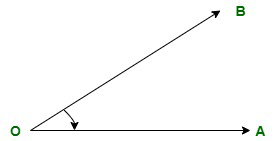Acute angle

In the figure, O is the vertex of the angle, and OA and OB are two intersecting rays that meet at point O forming an angle less than 90°. Hence, ∠AOB is an acute angle.

Right angle: The angle that exactly measures 90° is a right angle. It is also considered as a half straight angle as half of 180° makes a right angle. The value of the angle may be positive or negative on the basis of the rotation of the angle.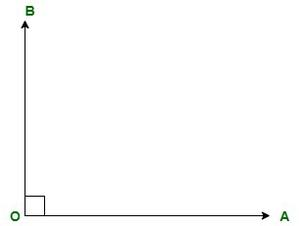Right angle

In the figure, O is the vertex of the angle, and OA and OB are two intersecting rays that meet at point O forming an angle of exactly 90°. Hence, ∠AOB is a right angle.

Obtuse angle: The angle that measures more than 90° and less than 180° is an obtuse angle. The degree always lies between 90° and 180°. The value of the obtuse angle will be positive if the rotation is anticlockwise and negative if the rotation is clockwise.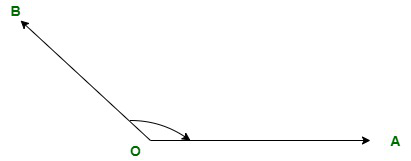Obtuse angle

In the figure, O is the vertex of the angle, and OA and OB are two intersecting rays that meet at point O forming an angle of more than 90°. Hence, ∠AOB is an obtuse angle.

Straight angle: The angle that measures exactly 180° is a straight angle. Straight angles form straight lines. The measure of straight angle can be positive or negative. If we move anticlockwise the straight angle will measure 180° and if we move clockwise the angle will measure -180°.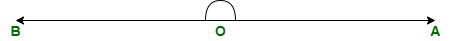Straight angle

In the figure, of a straight angle, we can observe that O is the meeting point of two arms that is called vertex and OA and OB are two sides of the angle.

Reflex angle: The angle that measures more than 180° and less than 360° is the reflex angle. The degree always lies between 180° and 360°.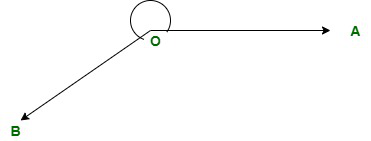Reflex angle

In the figure, O is the vertex of the angle, and OA and OB are two intersecting rays that meet at point O forming an angle of more than 180°. Hence, ∠AOB is a reflex angle.

Angles on the basis of rotation:

Positive angles: The angle that moves anticlockwise from its base and is drawn from the point (x, y) which is its origin is a positive angle.

Negative angles: The angle that moves clockwise from its base and is drawn from the point (-x, -y) which is its origin is a negative angle.

### What is a Zero Angle?

An angle that does not form a vertex or measure 0 degrees is called a zero angle. It is also called zero radians. A zero angle is formed when both the rays or arms of the angle are pointing towards the same direction and vertex as just a point without any space.

When we observe an angle it consists of a vertex and two intersecting rays. And in the case of zero angles, the vertex is a common point and two intersecting rays connected at the common point are coincided or merged with each other pointing towards the same direction.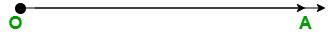zero angle

### Sample Questions

Question 1: Give a real-life example of zero angles?

The sunset at the horizon is a real-life example of zero angles.

Question 2: Is zero angle an acute angle?

Yes, zero angle is an acute angle as the range of acute angle measures from 0 degrees to less than 90 degrees.

Question 3: What are the five types of angles?

The five different angles are

1. Acute angle
2. Right angle
3. Straight angle
4. Obtuse angle
5. Reflex angle

Question 4: Are zero angle and complete angle the same?